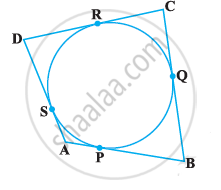Share

# a quadrilateral ABCD is drawn to circumscribe a circle, with centre O, in such a way that the sides AB, BC, CD and DA touch the circle at the points P, Q, R and S respectively. Prove that - CBSE Class 10 - Mathematics

ConceptNumber of Tangents from a Point on a Circle

#### Question

In the following Fig, a quadrilateral ABCD is drawn to circumscribe a circle, with centre O, in such a way that the sides AB, BC, CD and DA touch the circle at the points P, Q, R and S respectively. Prove that AB + CD = BC + DA.#### Solution

Since tangents drawn from an exterior point to a circle are equal in length,
AP = AS ….(1)

BP = BQ ….(2)

CR = CQ ….(3)

DR = DS ….(4)

Adding equations (1), (2), (3) and (4), we get

AP + BP + CR + DS = AS + BQ + CQ + DS

∴(AP + BP) + (CR + DR) = (AS + DS) + (BQ + CQ)

∴AB + CD = AD + BC

∴AB + CD = BC + DA …..(proved)

Is there an error in this question or solution?

#### Video TutorialsVIEW ALL 

Solution a quadrilateral ABCD is drawn to circumscribe a circle, with centre O, in such a way that the sides AB, BC, CD and DA touch the circle at the points P, Q, R and S respectively. Prove that Concept: Number of Tangents from a Point on a Circle.
S